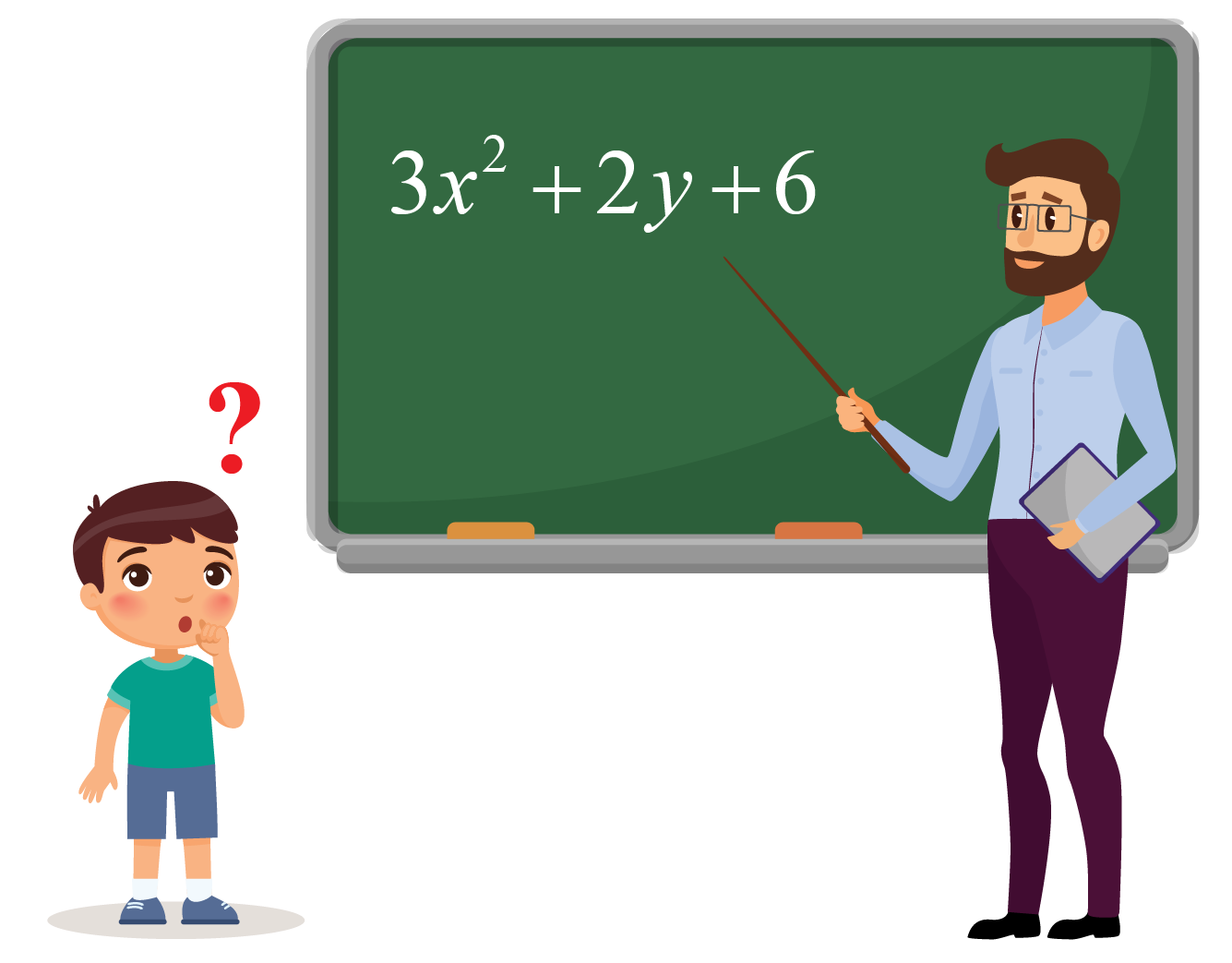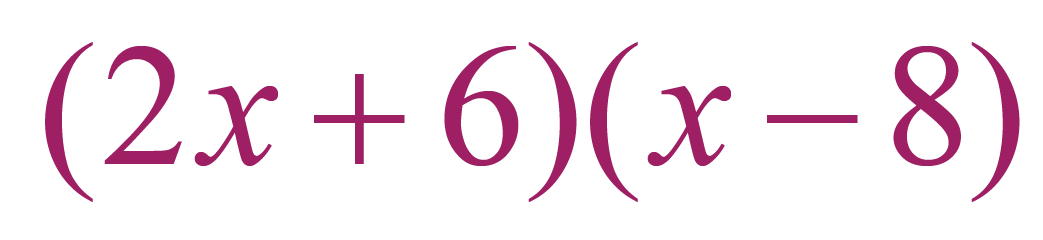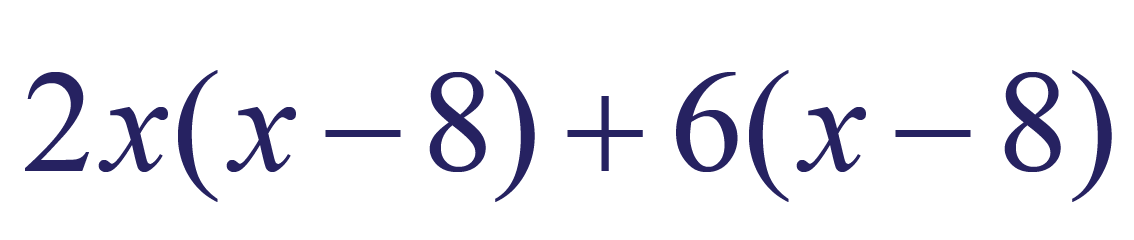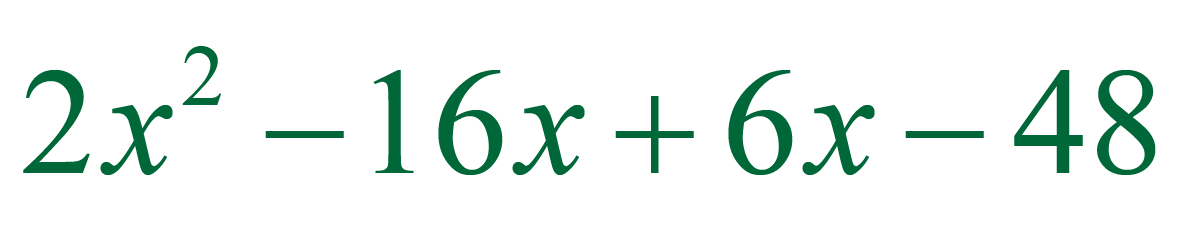# Polynomial Expressions

Polynomial Expressions
Go back to  'Polynomials'

In this mini lesson we will learn about polynomial expressions, degree of a polynomial, polynomial standard form, zero polynomial, polynomial expressions examples, and parts of a polynomial with solved examples and interactive questions.

The word polynomial is made of two words, "poly" which means 'many' and "nomial", which means terms. It was first used in the seventeenth century and is used in math for representing expressions.

Henry's teacher asked him whether the given expression was a polynomial expression or not?Do you want to know the answer?

## Lesson Plan

 1 What Are Polynomial Expressions? 2 Tips and Tricks 3 Important Notes on Polynomial Expressions 4 Solved Examples on Polynomial Expressions 5 Interactive Questions on Polynomial Expressions

## What Are Polynomial Expressions?

A polynomial is made up of terms, and each term has a coefficient while an expression is a sentence with a minimum of two numbers and at least one math operation in it.

The expressions which satisfy the criterion of a polynomial are polynomial expressions.

Let's see polynomial expressions examples in the following table.

### Examples

 Examples Polynomial Expressions or not $$x^2 + 3\sqrt{x} + 1$$ No $$x^2 + \sqrt{3}x + 1$$ Yes

In the two cases discussed above, the expression $$x^2 + 3\sqrt{x} + 1$$ is not a polynomial expression because the variable has a fractional exponent, i.e., $$\frac{1}{2}$$ which is a non-integer value; while for the second expression $$x^2 + \sqrt{3}x + 1$$, the fractional power $$\frac{1}{2}$$ is on the constant which is 3 in this case, hence it is a polynomial expression.

### Standard Form of Polynomial Expressions

The standard form of any polynomial expression is given when the terms of expression are ordered from the highest degree to the lowest degree.

The polynomial standard form can be written as:

$$a_{n}x^{n}+a_{n-1}x^{n-1}+.......+a_{2}x^2+a_{1}x+a_{0}$$

For example, $$ax^2 + bx + c$$.Tips and Tricks

• Positive powers associated with a variable are mandatory in any polynomial, thereby making them one among the important parts of a polynomial.
• Any expression having a non-integer exponent of the variable is not a polynomial. For example, $$\sqrt{x}$$ which has a fractional exponent.
• A polynomial expression should not have any square roots of variables, any fractional or negative powers on the variables, and no variables should be there in the denominators of any fractions.

## What Are the Types of Polynomials?

There are three types of polynomials based on the number of terms that they have:

• Monomial
• Binomial
• Trinomial

### Monomial

A monomial consists of only one term with a condition that this term should be non-zero.

Examples: $$6x$$, $$7x^3$$, $$2ab$$

### Binomial

A binomial is a polynomial that consists of two terms. It is written as the sum or difference of two or more monomials.

Examples: $$2x^4 + 8x$$, $$8y^3 + 3x$$, $$xy^2 + 3y$$

### Trinomial

A trinomial is a polynomial that consists of three terms.

Examples: $$3x^2 + 4x + 10$$, $$5y^4 + 3x^4 + 2x^2y^2$$, $$7y^2 + 3y + 17$$

## How to Find the Degree of a Polynomial Expression?

### For a Single Variable Polynomial

We find the degree of a polynomial expression using the following steps:

• Step 1: Combine the like terms of the polynomial expression.
• Step 2: Write the polynomial expression in the standard form.
• Step 3: Check and select the highest exponent.

The highest exponent of the expression gives the degree of a polynomial.

Let's consider the polynomial expression,

$$5x^3 + 4x^2 - x^4 - 2x^3 - 5x^2 + x^4$$

In this case, the expression can be simplified as,

$$3x^3 - x^2$$

Here, the highest exponent corresponding to the polynomial expression is 3

Hence, degree of polynomial expression is 3

### For a Multivariable Polynomial

If we take a polynomial expression with two variables, say x and y.

$$x^3 + 3x^2y^4 + 4y^2 + 6$$

We follow the above steps, with an additional step of adding the powers of different variables in the given terms.

Here, the degree of the polynomial is 6

This is because in $$3x^2y^4$$, the exponent values of x and y are 2 and 4 respectively.

When we add these, we get 6

Hence, the degree of the multivariable polynomial expression is 6

 Example 2

Which of the following polynomial expressions gives a monomial, binomial or trinomial on simplification? Give the answer in the standard form.

• $$2x^3 - 10x^3 + 12x^3$$
• $$2x^4 - 5x^3 + 9x^3 - 3x^4$$
• $$(x + 3)^2$$Solution

Jessica's approach to classify the polynomial expressions after classification would be as follows,

• $$2x^3 - 10x^3 + 12x^3$$

This expression on simplification gives, $$2x^3 - 10x^3 + 12x^3 = 4x^3$$

The obtained output is a single term which means it is a monomial.

• $$2x^4 - 5x^3 + 9x^3 - 3x^4$$

This expression on simplification gives, $$2x^4 - 5x^3 + 9x^3 - 3x^4 = 4x^3 - x^4$$

The obtained output has two terms which means it is a binomial.

In polynomial standard form the obtained expression is written as, $$(- x^4 + 4x^3)$$

• $$(x + 3)^2$$

The above expression can be simplified using algebraic identity of $$(a+b)^2$$

Hence, the above expression gives the value, $$x^2 - 6x + 9$$

The obtained output has three terms which means it is a trinomial.

The polynomial expression is in its standard form.

 $$\therefore$$ All the expressions are classified as monomial, binomial and polynomial.
 Example 3

How will Maria find the product of the polynomial expressions $$(2x+6)$$ and $$(x-8)$$?

Solution

She will write the product of the polynomial expressions as given below,Now to simplify the product of polynomial expressions, she will use the FOIL technique.

Using the distributive property, the above polynomial expressions can be written as,Now applying the FOIL technique, we get,Now combining the like terms we get,Hence, the product of polynomial expressions $$(2x+6)$$ and $$(x-8)$$ on simplification gives, $$(2x^2 - 10x - 48)$$

 $$\therefore$$ Maria simplified the product of polynomial expressions.

## Interactive Questions

Here are a few activities for you to practice.

## Let's Summarize

We hope you enjoyed understanding polynomial expressions and learning about polynomial, degree of a polynomial, polynomial standard form, zero polynomial, polynomial expressions examples, parts of a polynomial with the practice questions.

The mini-lesson targeted the fascinating concept of polynomial expressions. The math journey around polynomial expressions starts with what a student already knows, and goes on to creatively crafting a fresh concept in the young minds. Done in a way that not only it is relatable and easy to grasp, but also will stay with them forever. Here lies the magic with Cuemath.

At Cuemath, our team of math experts is dedicated to making learning fun for our favorite readers, the students!

Through an interactive and engaging learning-teaching-learning approach, the teachers explore all angles of a topic.

Be it worksheets, online classes, doubt sessions, or any other form of relation, it’s the logical thinking and smart learning approach that we at Cuemath believe in.

## FAQs on Polynomial Expressions

### 1. What is a polynomial expression?

Any expression which is a polynomial is called a polynomial expression.

### 2. How do you know if an expression is a polynomial?

If an expression satisfies two criteria:

• Only the operations of addition, subtraction, multiplication and division by constants is done.
• The exponents of the variables are non-negative integers.

Polynomials
Grade 9 | Questions Set 1
Polynomials
Polynomials
Grade 10 | Questions Set 1
Polynomials